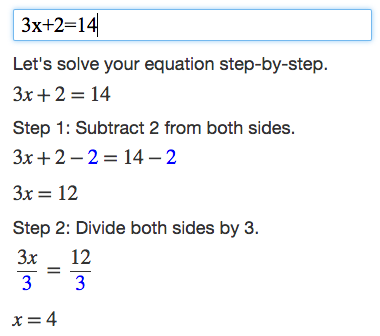# Algebra Calculator

#### What do you want to calculate?

Examples: 1+2, 1/3+1/4, 2^3 * 2^2
(x+1)(x+2) (Simplify Example), 2x^2+2y @ x=5, y=3 (Evaluate Example)
y=x^2+1 (Graph Example), 4x+2=2(x+6) (Solve Example)

Algebra Calculator is a calculator that gives step-by-step help on algebra problems.

## How to Use the Calculator

Type your algebra problem into the text box.

For example, enter 3x+2=14 into the text box to get a step-by-step explanation of how to solve 3x+2=14.### More Examples

Trying the examples on the Examples page is the quickest way to learn how to use the calculator.

### Math Symbols

If you would like to create your own math expressions, here are some symbols that the calculator understands:

- (Subtraction)
* (Multiplication)
/ (Division)
^ (Exponent: "raised to the power")
sqrt (Square Root) (Example: sqrt(9))

More Math Symbols

### Tutorial

Read the full tutorial to learn how to graph equations and check your algebra homework.

### Mobile App

Get the MathPapa mobile app! It works offline!